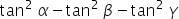Maths-
General
Easy

###Hint:

In this question, we have to find the value of. For that first we will use the given equation to find the value of one of the three term, then we can put the value obtained into find the required answer.

## Book A Free Demo+91